# Force and Pressure

## Objective Questions

#### Question 1

Write true or false for each statement:

(a) The S.I. unit of force is kgf.

(b) A force always produces both the linear and turning motions.

(c) Moment of force = force x perpendicular distance of force from the pivoted point.

(d) Less force is needed when applied at a farther distance from the pivoted point.

(e) For a given thrust, pressure is more on a surface of large area.

(f) The pressure on a surface increases with an increase in the thrust on the surface.

(g) A man exerts same pressure on the ground whether he is standing or he is lying.

(h) It is easier to hammer a blunt nail into a piece of wood than a sharply pointed nail.

(i) The S.I. unit of pressure is pascal.

(j) Water in a lake exerts pressure only at its bottom.

(k) A liquid exerts pressure in all directions.

(l) Gases exert pressure in all directions.

(m) The atmospheric pressure is nearly 105 Pa.

(n) Higher we go, greater is the air pressure.

(a) False
Correct Statement — The S.I. unit of force is newton.

(b) False
Correct Statement — A force can produce either linear motion or turning motion.

(c) True

(d) True

(e) False
Correct Statement — Pressure is defined as thrust per unit area. Hence, for a given thrust, pressure is less on a surface of large area.

(f) True

(g) False
Correct Statement — A man exerts more pressure while standing than lying on the ground as area of contact is less in case of standing as compared to lying on the ground.

(h) False
Correct Statement — Incase of blunt nail, the thrust exerted on hammering acts on a larger area so less pressure acts on the piece of wood making it difficult to hammer the nail.

(i) True

(j) False
Correct Statement — Water in a lake exerts pressure in all directions.

(k) True

(l) True

(m) True

(n) False
Correct Statement — Higher we go, lesser is the air pressure.

#### Question 2

Fill in the blanks:

(a) 1 kgf = ............... N (nearly).

(b) Moment of force = ............... x distance of force from the point of turning.

(c) In a door, handle is provided ............... from the hinges.

(d) The unit of thrust is ............... .

(e) Thrust is the ............... force acting on a surface.

(f) Pressure is the thrust acting on a surface of ............... area.

(g) The unit of pressure is ............... .

(h) Pressure is reduced if ............... increases.

(i) Pressure in a liquid ............... with the depth.

(j) The atmospheric pressure on earth surface is nearly ............... .

(a) 10

(b) Force

(c) Farthest

(d) newton

(e) normal

(f) unit

(g) pascal

(h) surface area

(i) increases

(j) 105 Pa

#### Question 3

Match the following:

Column AColumn B
(a) Camel(i) broad and deep foundation
(c) Knife(iii) six or eight tyres
(d) High building(iv) sharp cutting edge
(e) Thrust(v) atm
(f) Moment of force(vi) N
(g) Atmospheric pressure(vii) N m

Column AColumn B
(b) Truck(iii) six or eight tyres
(c) Knife(iv) sharp cutting edge
(d) High building(i) broad and deep foundation
(e) Thrust(vi) N
(f) Moment of force(vii) N m
(g) Atmospheric pressure(v) atm

#### Question 4a

Select the correct alternative:

S.I. unit of moment of force is:

1. N
2. N cm
3. kgf m
4. N m

N m

Reason — S.I. Unit of moment of force = S.I. Unit of force x S.I. Unit of distance = N x m
So unit of moment of force = N m.

#### Question 4b

Select the correct alternative:

To obtain a given moment of force for turning a body, the force needed can be decreased by:

1. applying the force at the pivoted point.
2. applying the force very close to the pivoted point.
3. applying the force farthest from the pivoted point.
4. none of the above.

Applying the force farthest from the pivoted point.

Reason — Larger the perpendicular distance of the point at which the force is applied from the pivoted point, less force is needed to obtain a given moment of force for turning a body.

#### Question 4c

Select the correct alternative:

The unit of thrust is:

1. kgf
2. kg
3. g
4. m s-1

kgf

Reason — The gravitational unit of thrust is kgf.

#### Question 4d

Select the correct alternative:

The unit of pressure is:

1. N x m
2. kgf
3. N m-2
4. kgf m2

N m-2

Reason

Unit of Pressure = $\dfrac{\text{Unit of Force}}{\text{Unit of Area}}$ = $\dfrac{\text{N}}{\text m^2}$

So, unit of pressure is N m-2

#### Question 4e

Select the correct alternative:

The pressure and thrust are related as:

1. Pressure = Thrust
2. Pressure = Thrust x Area
3. Pressure = $\dfrac{\text{Thrust}}{\text{Area}}$
4. Pressure = $\dfrac{\text{Area}}{\text{Thrust}}$

Pressure = $\dfrac{\text{Thrust}}{\text{Area}}$

Reason — Pressure is defined as thrust acting per unit area.

#### Question 4f

Select the correct alternative:

A body weighing 5 kgf, placed on a surface of area 0.1 m2, exerts a thrust on the surface equal to:

1. 50 kgf
2. 5 kgf
3. 50 kgf m-2
4. 5 kgf m-2

5 kgf

Reason — A body when placed on a surface exerts a thrust on the surface which is equal to its own weight. So thrust = weight of the body = 5 kgf.

#### Question 4g

Select the correct alternative:

The feet of lizards act like:

4. none of the above

Reason — Lizards can stay and move on walls because their feet behave like suction pads so they remain pressed against the wall due to atmospheric pressure.

#### Question 4h

Select the correct alternative:

Pressure exerted by a liquid is due to its:

1. weight
2. mass
3. volume
4. area

Weight

Reason — Liquids have weight and pressure is weight acting per unit area.

#### Question 4i

Select the correct alternative:

Pressure inside a liquid increases with:

1. increase in depth.
2. decrease in depth.
3. decrease in density.
4. none of the above.

increase in depth

Reason — Pressure of liquid at a point increases with the height of the liquid column above it, so as depth increases pressure inside liquid increases.

#### Question 4j

Select the correct alternative:

The atmospheric pressure at sea level is nearly:

1. 10 Pa
2. 100,000 Pa
3. 100 Pa
4. 10,000 Pa

100,000 Pa

Reason — At sea level, the atmospheric pressure is 100,000 Pa which is 76 cm of mercury column.

#### Question 4k

Select the correct alternative:

Nose bleeding may occur at a high altitude because:

1. the atmospheric pressure decreases.
2. the oxygen content of atmosphere decreases.
3. the atmospheric pressure increases.
4. there are strong air currents at the high altitude.

the atmospheric pressure decreases

Reason — At higher altitudes, atmospheric pressure decreases but our body pressure remains the same. So, inside pressure becomes more than atmospheric pressure which causes nose bleeding.

#### Question 1

Define force. State its S.I. unit.

Force is that cause which changes the state of the body (either the state of rest or the state of motion) or changes the size or shape of the body. The S.I. unit of force is newton (N).

#### Question 2

State two effects of a force when applied on a body.

The two effects of a force when applied on a body are —

(i) It can change the shape or size of a body.

(ii) It can stop a moving body or it can move a stationary body.

#### Question 3

How does the effect of a force differ when it is applied on

(a) a rigid body

(b) a non-rigid body?

(a) When a force is applied on a rigid body, it produces only change in motion of the body. The force does not cause any change in the inter-spacing between its constituent particles.

(b) When a force is applied on a non-rigid body, it causes both change in its size or shape and motion in it.

#### Question 4

State the effect of force F in each of the following diagrams (a) and (b).(i) In figure (a), when a force F is applied on the ball, the ball starts moving in a straight line in the direction of force F and continues to move in that direction. This is called linear motion.(ii) In figure (b), when a force F is applied on the wheel pivoted at a point, the force turns the wheel in anticlockwise direction about the axis of rotation producing turning motion.#### Question 5

Define the term moment of force.

The moment of force is equal to the product of the magnitude of the force and the perpendicular distance of the force from the pivoted point.

#### Question 6

State the S.I. unit of moment of force.

The S.I. unit of moment of force is newton x metre (N m).

#### Question 7

State two factors which affect moment of force.

The two factors which affect moment of force are —

(i) Magnitude of force.

(ii) Perpendicular distance of force from the pivoted point.

#### Question 8

In figure below, a force F is applied in a direction passing through the pivoted point O of the body. Will the body rotate? Give reason to support your answer.No, the body will not rotate because
Turning effect = Force x perpendicular distance of the force from the pivoted point.
= F x 0 = 0.
Perpendicular distance is 0 or force is parallel to the point of application of force, hence the body will not rotate.

#### Question 9

Write the expression for the moment of force about a given axis of rotation.

The expression for the moment of force about a given axis of rotation is —

Moment of force about a given axis = Force x perpendicular distance of force from the axis of rotation.

#### Question 10

State one way to decrease the moment of a given force about a given axis of rotation.

The moment of given force about a given axis of rotation is decreased by either decreasing the force or by decreasing the perpendicular distance of force from the axis of rotation.

#### Question 11

State one way to obtain greater moment of a given force about a given axis of rotation.

One way to obtain greater moment of a given force is to increase the distance from the axis of rotation where the force would act.

#### Question 12

What do you mean by the clockwise and anti-clockwise moment of force?

If the effect on the body is to turn it clockwise, the moment of force is called clockwise moment. If the effect on the body is to turn it anti-clockwise, the moment of force is called anti-clockwise moment.

#### Question 13(a)

Explain the following:

The spanner (or wrench) has a long handle.

A spanner has a long handle to produce a large turning effect by applying a small amount of force at the end of the handle as shown in the figure below: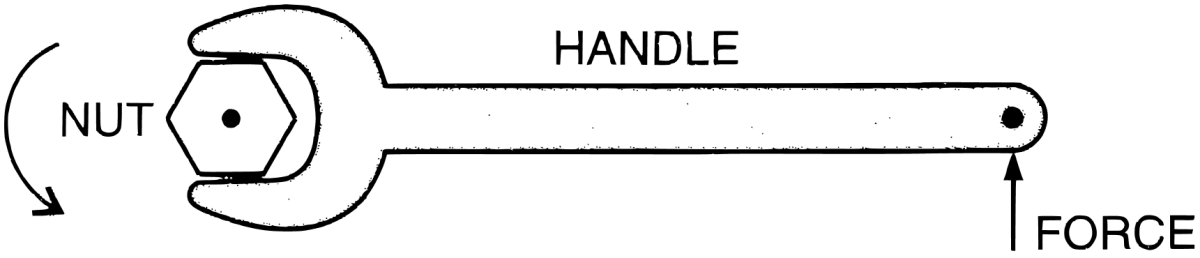#### Question 13(b)

Explain the following:

The steering wheel of a vehicle is of large diameter.

It is easier to turn the steering wheel of large diameter as larger the diameter, larger is the perpendicular distance from the axis of rotation so less force is applied to generate same amount of turning effect as compared to steering wheel of small diameter.

#### Question 13(c)

Explain the following:

The hand flour grinder is provided with a handle near the rim.

The hand flour grinder is provided with a handle near the rim which is at a maximum distance from the axis of rotation so that it can easily be rotated at its centre by applying a small force at the handle.

#### Question 13(d)

Explain the following:

It is easier to open the door by pushing it at its free end.

It is easier to open the door by pushing it at its free end because larger the perpendicular distance from the axis of rotation, less is the force required to open it.

#### Question 13(e)

Explain the following:

A potter turns his wheel by applying a force through the stick near the rim of wheel.

A potter's wheel is pivoted at the centre. When the potter applies a force through the stick near the rim of the wheel, the point of application of force is at a maximum distance from the axis of rotation, hence less force is required to rotate the wheel.

#### Question 14

What is thrust?

If a force is applied on a surface in a direction perpendicular to the surface, the force is called thrust. In other words, thrust is the force acting normally on the surface.

#### Question 15

State the unit of thrust.

The units of thrust are kilogram force(kgf), gram force(gf) and newton(N) (same as that of weight or force).

#### Question 16

On what factors does the effect of thrust on a surface depend?

The effect of thrust depends on the area of the surface on which it acts. Smaller the area of the surface on which a thrust acts larger is its effect and effect of thrust is less on a larger surface area.

#### Question 17

Define the term pressure and state its unit.

Pressure is defined as the thrust acting per unit area.

Pressure = $\dfrac{\text{Thrust}}{\text{Area}}$

Its unit is pascal (Pa) or $\dfrac{\text{newton}}{\text{metre}^2}$ (N m-2).

#### Question 18

How is thrust related to pressure?

Pressure is thrust acting per unit area. Thrust is directly proportional to pressure. Greater the thrust, greater is the pressure and smaller the thrust, smaller is the pressure.
Pressure = $\dfrac{\text{Thrust}}{\text{Area}}$

#### Question 19

Name two factors on which the pressure on a surface depends.

The two factors on which the pressure on a surface depends are:

1. Area of the surface on which thrust acts.
2. Magnitude of thrust acting on the surface.

#### Question 20

When does a man exert more pressure on the floor: while standing or while walking?

A man exerts more pressure on the surface while walking than standing because while walking one foot is in contact with the floor so surface area is less but while standing both foot are in contact with the floor providing a large surface area. Since pressure is inversely proportional to surface area so pressure exerted while walking is more as compared to pressure exerted on standing.

#### Question 21

Why do camels or elephants have broad feet?

The broad feet of camels and elephants increases the surface of the feet in contact with the ground. This reduces the pressure exerted on the ground as pressure is inversely proportional to surface area.
Thus, this helps camels to walk easily on the desert sand without their feet sinking in it. The large body weight of elephants is supported by their broad feet helping them to move around comfortably.

#### Question 22

A sharp pin works better than a blunt pin. Explain the reason.

A sharp pin has less surface area as compared to a blunt pin, so for a given applied force sharp pin exerts more pressure than blunt pin and penetrates a surface like wood or wall easily. So, it works better than a blunt pin.

#### Question 23

Why is the bottom part of the foundation of a building made wider?

The bottom part of the foundation of a building is made wider so that the weight of the building will act on larger surface area exerting less pressure on the ground (as pressure is inversely proportional to surface area). This avoids sinking of buildings into the earth.

#### Question 24

It is easier to cut with a sharp knife than with a blunt one. Explain.

A sharp knife has sharp edges that provides less surface area of contact as compared to a blunt knife which has more surface area of contact. So pressure applied by the force is more in sharp knife which makes it easier to cut than a blunt one.

#### Question 25

A gum bottle rests on its base. If it is placed upside down,

(i) How does the thrust change?

(ii) How does the pressure change?

(i) A gum bottle has broader base and narrower neck and when it is placed upside down there will be no change in thrust as thrust is the weight of the body that is exerted on the surface.

(ii) When gum bottle is placed upside down, surface area is less so pressure will be more as pressure is inversely proportional to surface area.

#### Question 26

Explain the following:

(i) Sleepers are used below the rails.

(ii) A tall building has wide foundations.

(i) Wide wooden sleepers are placed below the railway tracks to increase the surface area of the tracks in contact with the ground. This reduces the pressure exerted by the rails on the ground.

(ii) The foundation of a tall building is made wider because the weight of the building will act on larger surface area exerting less pressure on the ground (as pressure is inversely proportional to surface area). This avoids sinking of building into the earth.

#### Question 27

Describe an experiment to show that a liquid exerts pressure at the bottom of the container in which it is kept.

The following experiment demonstrates that a liquid exerts pressure at the bottom of the container in which it is kept:1. Take a glass tube and tie a balloon at its lower end.
2. Hold it vertically straight as shown in the above figure.
3. Pour some water in the tube.

Observations — After sometime we will notice that the balloon bulges out as shown in figure (b) above.

Conclusion — Balloon bulges out because water column exerts pressure at its bottom. The force on the balloon is equal to the weight of the water column known as thrust.
So, Pressure = $\dfrac{\text{Thrust}}{\text{Area}}$ = $\dfrac{\text{W}}{\text{A}}$, where W is weight of the water column (thrust) and A is surface area.

#### Question 28

Describe a suitable experiment to demonstrate that a liquid exerts pressure sideways also.

The following experiment demonstrates that a liquid exerts pressure sideways also:1. Take a glass tube closed at one end and having an opening in its side near the bottom.
2. Tie a deflated balloon at the side opening of the tube.
3. Hold the tube vertically straight as shown in the above figure.
4. Pour some water in the tube.

Observations — After sometime we will notice that the balloon bulges out as shown in figure (b) above.

Conclusion — From the above experiment it is concluded that a liquid exerts pressure sideways also.

#### Question 29

Describe a simple experiment to show that at a given depth, a liquid exerts same pressure in all directions.

The following experiment demonstrates that at a given depth, a liquid exerts same pressure in all directions: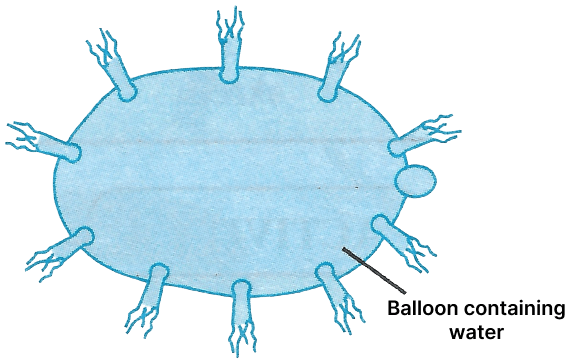1. Take a balloon and fill it with water.
2. Tie the mouth of the balloon.
3. Make holes in the balloon by inserting pins at several places in all directions.

Water comes out through each hole which shows that liquid exerts same pressure in all directions.

#### Question 30

State two factors on which the pressure at a point in a liquid depends.

The two factors on which the pressure at a point in a liquid depends are:

1. The height of the liquid column — Liquid pressure increases with the height of the liquid column above the point.
2. The density of the liquid — Liquid pressure increases with the increase in the density of the liquid.

#### Question 31

Describe an experiment to show that the liquid pressure at a point increases with the increase in height of the liquid column above that point.

The experiment is described below:1. Take a glass tube open at both ends. Hold it vertically.
2. Tie a balloon at its lower end.
3. Pour some water in the tube.
4. Balloon starts bulging out as shown in figure (a) above.
5. Add more water in the tube.
6. Balloon will bulge more as shown in figure (b) above.

Conclusion — From the above experiment it is concluded that when the height of the water column increases, more pressure is exerted on the balloon and it bulges out more. This shows that the liquid pressure at a point increases with the increase in height of the liquid column above that point.

#### Question 32

Which fact about liquid pressure does the diagram in figure below illustrate?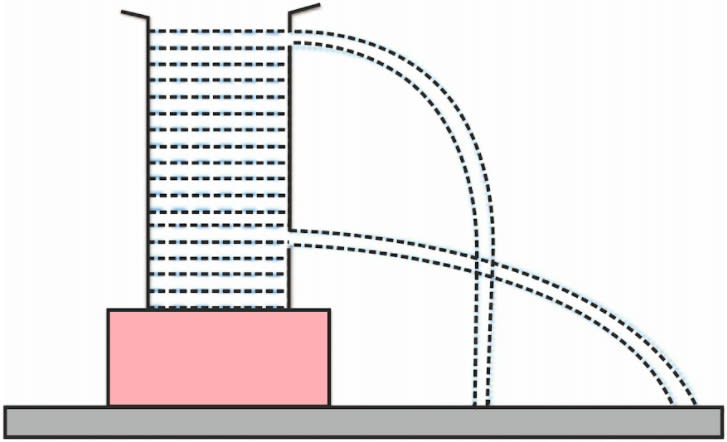The diagram demonstrates that liquid pressure at a point increases with the height of the liquid column above it as water flowing out from upper hole falls near the cylinder while water from lower hole falls far from the cylinder.

#### Question 33

Describe an experiment to show that liquid pressure depends on the density of liquid.

The following experiment demonstrates that liquid pressure depends on the density of liquid: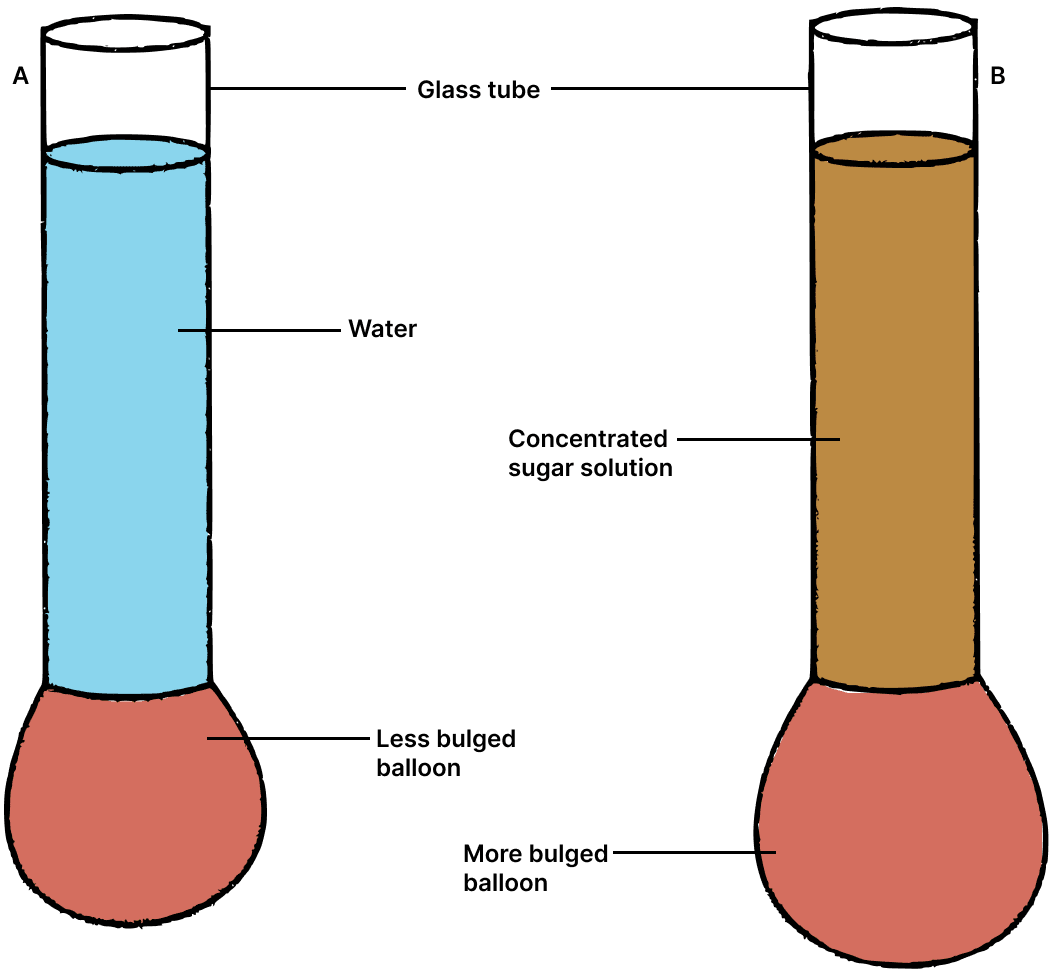1. Take two identical glass tubes open at both the ends. Mark them A and B.
2. Hold both the tubes vertically and tie an inflated balloon to both the tubes at their lower ends.
3. In tube A pour some water.
4. In tube B pour some concentrated sugar solution such that its height is same as that of height of water in tube A.

Observation — It is noticed that the balloon attached to tube B bulges out more as compared to balloon attached to tube A as shown in the above figure.

Conclusion — Density of sugar solution is more than water so it exerts more pressure which shows that liquid pressure depends on the density of liquid.

#### Question 34

A dam has broader walls at the bottom than at the top. Give a reason.

The pressure at a point due to a liquid increases with the increase in height of the liquid column above it, so to withstand the increasing pressure of water a dam has broader walls at the bottom than at the top.

In the figure given below, the increasing length of arrows in water represents the increasing pressure on the wall of the dam towards the bottom.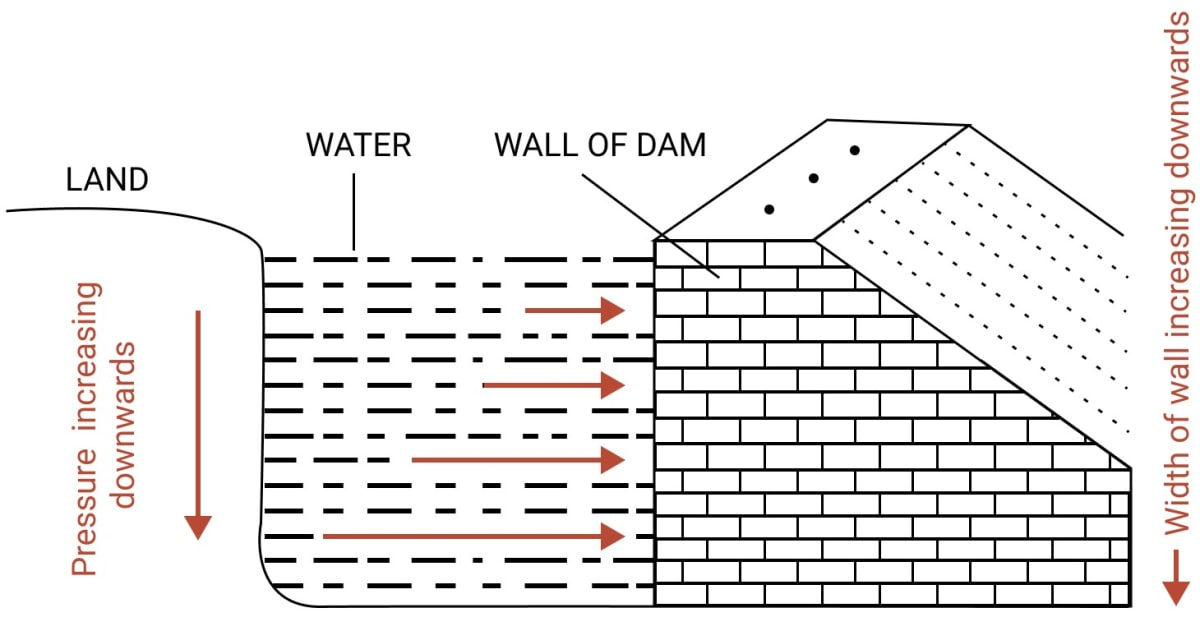#### Question 35

What do you mean by atmospheric pressure?

The thrust on unit area of the earth surface due to the column of air is called the atmospheric pressure.

#### Question 36

Write the numerical value of the atmospheric pressure on the earth surface in pascal.

The atmospheric pressure on the earth surface in pascal is 1.013 x 105 Pa.

#### Question 37

We do not feel uneasy even under the enormous atmospheric pressure. Give a reason.

The blood in the veins of our body exerts a pressure which is slightly more than the atmospheric pressure which makes the effect of atmospheric pressure ineffective. So we do not feel uneasy even under the enormous atmospheric pressure.

#### Question 38

Describe a simple experiment to illustrate that air exerts pressure.

The following experiment demonstrates that air exerts pressure: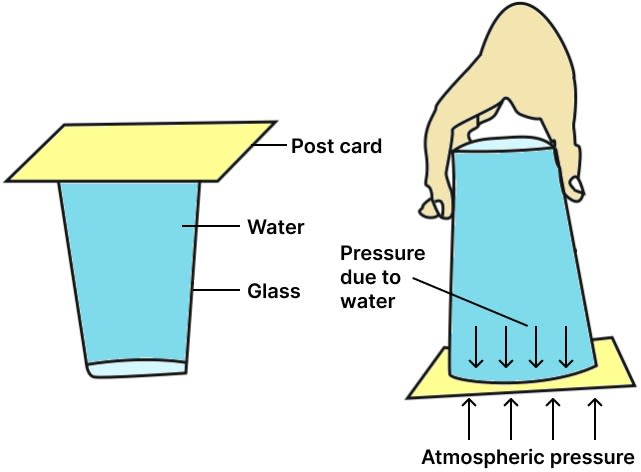1. Take a glass filled with water up to its brim and place a post card on top of it.
2. Press the palm of your one hand on top of post card and then invert the filled glass upside down.
3. Now gently remove your hand from the post card to release it.

Conclusion — It is observed that post card does not fall from the glass because the atmospheric pressure is acting upwards on the post card from outside the glass which overcomes the pressure on post card due to water in the glass.

#### Question 39

Describe the crushing tin can experiment. What do you conclude from this experiment?

Crushing tin can experiment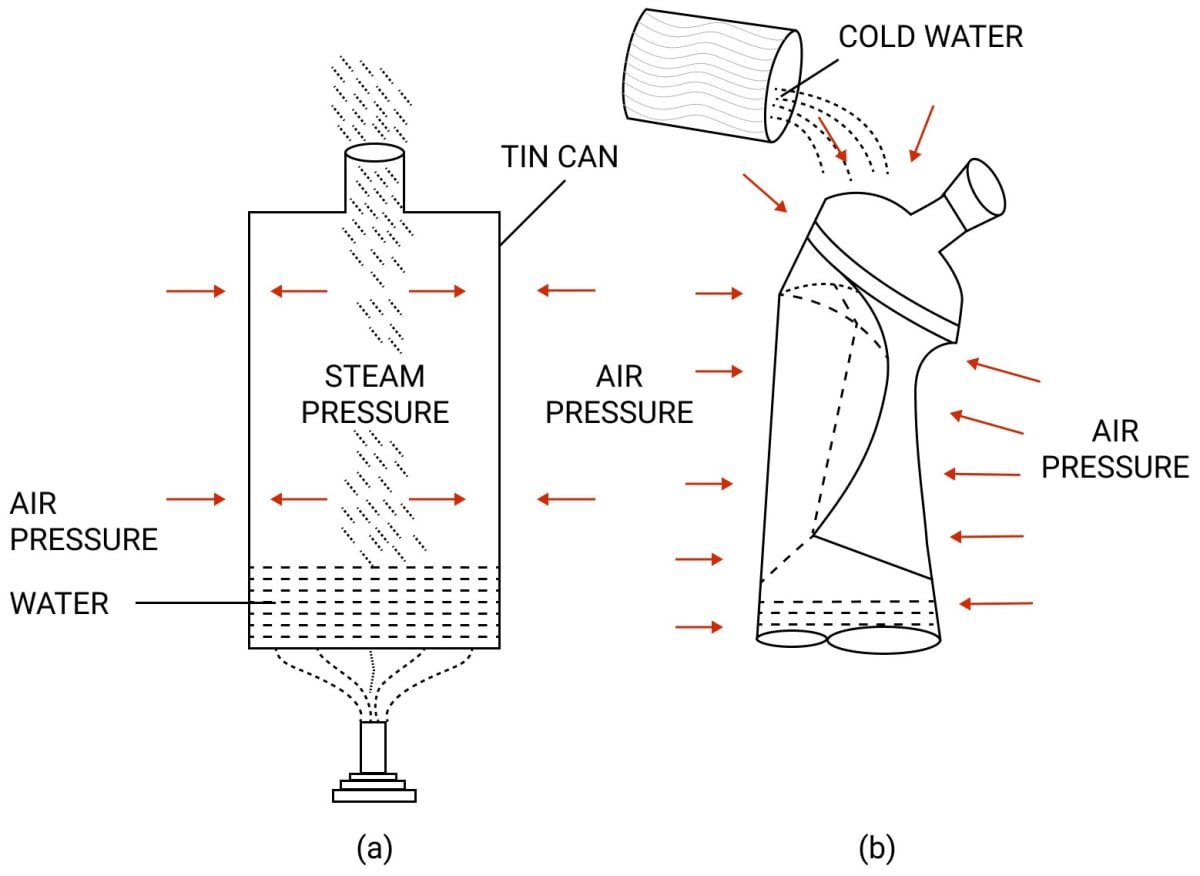1. Take a thin walled tin can with airtight stopper. Remove the stopper.
2. Fill the can partially with water. Heat the can over the flame of a burner till water boils.
3. Now the air pressure inside and outside the can is same.
4. When steam comes out of the opening put the stopper.
5. Remove the can from the burner.
6. Place the can in the tub and pour cold water on the can.

It is observed that the can collapses. The reason is that when steam comes out it takes away most of the air from the can. When cold water is poured on the can, steam condenses into water leaving a partial vacuum in the can. The air pressure outside is more than inside which exerts force on the can causing it to collapse.

From this experiment it is concluded that air exerts pressure.

#### Question 40(a)

Give reasons for the following:

A balloon collapses when air is removed from it.

When air is removed from the balloon, the pressure inside it becomes much less than the outside atmospheric pressure and hence the balloon collapses.

#### Question 40(b)

Give reasons for the following:

Water does not run out of a dropper unless its rubber bulb is pressed.

There are two forces acting on the water inside the dropper one is liquid pressure from inside and atmospheric pressure from outside. Atmospheric pressure acting from outside balances the liquid pressure from inside so water does not come out from the dropper. When bulb is pressed the liquid pressure increases than atmospheric pressure and water comes out from the dropper.

#### Question 40(c)

Give reasons for the following:

Two holes are made in a sealed oil tin to take out oil from it.

Two holes are made in a sealed oil tin to take out oil from it because through one hole atmospheric pressure acts due to air entering through it and through another hole the oil comes out easily.

#### Question 41

How does the atmospheric pressure change with altitude?

The atmospheric pressure decreases with increase in altitude. So, as we go higher the atmospheric pressure decreases.

## Numericals

#### Question 1

Find the moment of force of 20 N about an axis of rotation at a distance of 0.5 m from the force.

Given:
Force f = 20 N
Distance d = 0.5 m
Moment of force = ?

Moment of force = force f x distance d
= 20 x 0.5
= 10 N m

So, the moment of force = 10 N m.

#### Question 2

The moment of a force of 25 N about a point is 2.5 N m. Find the perpendicular distance of force from that point.

Given:
Force f = 25 N
Moment of force = 2.5 N m
Perpendicular distance d = ?

$\text{Moment of force} = \text{force f} \times \text{distance d} \\[1em] \text{Distance d}= \dfrac{\text{Moment of force}}{\text{Force f}} \\[1em] = \dfrac{\text{2.5}}{\text{25}} \\[1em] = 0.1 \text{ m}$

So, perpendicular distance of force from the point = 0.1 m or 10 cm.

#### Question 3

A spanner of length 10 cm is used to unscrew a nut by applying a minimum force of 5.0 N. Calculate the moment of force required.

Given:
Force f = 5.0 N
Distance d = 10 cm = 0.1 m
Moment of force = ?

Moment of force = force f x distance d
= 5.0 x 0.1
= 0.5 N m

So, Moment of force = 0.5 N m.

#### Question 4

A wheel of diameter 2 m can be rotated about an axis passing through its centre by a moment of force equal to 2.0 N m. What minimum force must be applied on its rim?

Given:
Moment of force = 2.0 N m
Diameter = 2 m so radius = 1 m
Therefore perpendicular distance d = radius = 1 m
Force f = ?

$\text{Moment of force} = \text{force f} \times \text{distance d} \\[1em] \text{Force f}= \dfrac{\text{Moment of force}}{\text{distance d}} \\[1em] = \dfrac{\text{2.0}}{\text{1}} \\[1em] = 2 \text{ N}$

So, minimum force required is 2 N.

#### Question 5

A normal force of 200 N acts on an area 0.02 m2. Find the pressure in pascal.

Given:
Force (Thrust) f = 200 N
Area A = 0.02 m2
Pressure = ?

$\text{Pressure} = \dfrac{\text{Thrust}}{\text{Area}} \\[1em] = \dfrac{\text{200}}{\text{0.02}} \\[1em] = 10000 \text{ Pa}$

So Pressure = 10000 Pa.

#### Question 6

Find the thrust required to exert a pressure of 50,000 Pa on an area of 0.05 m2.

Given:
Pressure = 50000 Pa
Area = 0.05 m2
Thrust = ?

Pressure = $\dfrac{\text{Thrust}}{\text{Area}}$

Thrust = Pressure x Area = 50000 x 0.05 = 2500 N

So, Thrust = 2500 N.

#### Question 7

Find the area of a body which experiences a pressure of 50,000 Pa by a thrust of 100 N.

Given:
Pressure = 50,000 Pa
Thrust = 100 N
Area = ?

$\text{Pressure}= \dfrac{\text{Thrust}}{\text{Area}} \\[1em] \text{Area}= \dfrac{\text{Thrust}}{\text{Pressure}} \\[1em] = \dfrac{100}{50000} \\[1em] = 0.002 \text{ m}^{2}$

So Area of body = 0.002 m2 or 2 x 10-3 m2.

#### Question 8

Calculate the pressure in pascal exerted by a force of 300 N acting normally on an area of 30 cm2.

Given:
Force (Thrust) = 300 N
Area = 30 cm2 = $\dfrac{30}{10000}$ = 0.003 m2
Pressure = ?

$\text{Pressure}= \dfrac{\text{Thrust}}{\text{Area}} \\[1em] = \dfrac{300}{0.003} \\[1em] = 1,00,000 \text{ Pa}$

So Pressure = 1,00,000 Pa or 105 Pa.

#### Question 9

How much thrust will be required to exert a pressure of 20,000 Pa on an area of 1 cm2?

Given:
Pressure = 20000 Pa
Area = 1 cm2 = $\dfrac{\text{1}}{\text{10000}}$ = 0.0001 m2 or 10-4 m2
Thrust = ?

Pressure = $\dfrac{\text{Thrust}}{\text{Area}}$
Thrust = Pressure x Area
= 20000 x 10-4
= 2 N

So Thrust = 2 N.

#### Question 10

The base of a container measures 15 cm x 20 cm. It is placed on a table top. If the weight of the container is 60 N, what is the pressure exerted by the container on the table top?

Given:
Weight = Thrust = 60 N
Area = 15 cm x 20 cm = 300 cm2 = $\dfrac{\text{300}}{\text{10000}}$ = 0.03 m2
Pressure = ?

$\text{Pressure}= \dfrac{\text{Thrust}}{\text{Area}} \\[1em] = \dfrac{\text{60}}{\text{0.03}} \\[1em] = 2000 \text{ Pa}$

So, Pressure = 2000 Pa.

#### Question 11

Calculate the pressure exerted on a surface of 0.5 m2 by a thrust of 100 kgf.

Given:
Thrust = 100 kgf
Area = 0.5 m2
Pressure = ?

$\text{Pressure}= \dfrac{\text{Thrust}}{\text{Area}} \\[1em] = \dfrac{\text{100}}{\text{0.5}} \\[1em] = 200 \text{ kgf m}^{-2}$

So Pressure = 200 kgf m-2.

#### Question 12

A boy weighing 60 kgf stands on a platform of dimensions 2.5 cm x 0.5 cm. What pressure in pascal does he exert?

Given:
Thrust = Weight = 60 kgf
1 kgf = 10 N
∴ 60 kgf = 60 x 10 = 600 N
So, Thrust = 600 N

Area = 2.5 cm x 0.5 cm = 1.25 cm2 = $\dfrac{\text{1.25}}{\text{10000}}$ = 0.000125 m2
Pressure =?

$\text{Pressure}= \dfrac{\text{Thrust}}{\text{Area}} \\[1em] = \dfrac{\text{600}}{\text{0.000125}} \\[1em] = 48,00,000 \text{ Pa}$

So, Pressure = 48,00,000 or 4.8 x 106 Pa.

#### Question 13

Figure below shows a brick of weight 2 kgf and dimensions 20 cm x 10 cm x 5 cm placed in three different positions on the ground. Find the pressure exerted by the brick in each case.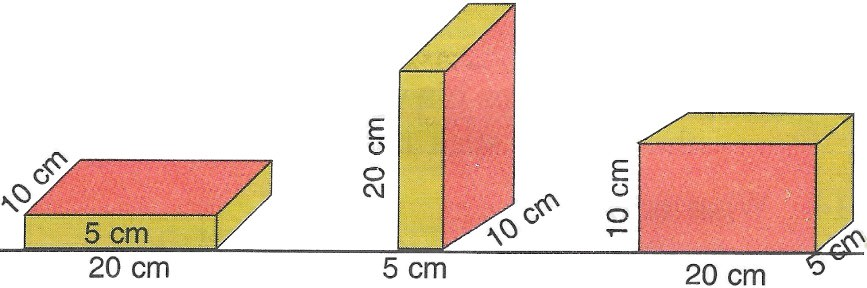First case:

Thrust = Weight = 2 kgf
Area = 20 cm x 10 cm = 200 cm2
Pressure = ?

$\text{Pressure}= \dfrac{\text{Thrust}}{\text{Area}} \\[1em] = \dfrac{\text{2}}{\text{200}} \\[1em] = 0.01 \text{ kgf cm}^{-2}$

So Pressure exerted by brick in first figure is 0.01 kgf cm-2.

Second case:

Thrust = Weight = 2 kgf
Area = 5 cm x 10 cm = 50 cm2
Pressure = ?

$\text{Pressure}= \dfrac{\text{Thrust}}{\text{Area}} \\[1em] = \dfrac{\text{2}}{\text{50}} \\[1em] = 0.04 \text{ kgf cm}^{-2}$

So Pressure exerted by brick in second figure is 0.04 kgf cm-2.

Third case:

Thrust = Weight = 2 kgf
Area = 20 cm x 5 cm = 100 cm2
Pressure = ?

$\text{Pressure}= \dfrac{\text{Thrust}}{\text{Area}} \\[1em] = \dfrac{\text{2}}{\text{100}} \\[1em] = 0.02 \text{ kgf cm}^{-2}$

So, Pressure exerted by brick in third figure is 0.02 kgf cm-2.